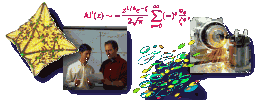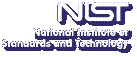Up# High spatial order vortex methods and Lagrangian techniques using deforming basis functions

Louis Rossi
Department of Mathematical Sciences, University of Delaware

Tuesday, May 20, 2003 15:00-16:00,
Room 145, NIST North (820)
Gaithersburg
Tuesday, May 20, 2003 13:00-14:00,
Room 4550
Boulder

Abstract: Broad classes of numerical schemes known as vortex methods, particle tracking methods, and smoothed particle hydrodynamics all exhibit the common theme of approximating solutions to linear and nonlinear partial differential equations as a linear combination of moving, localized basis functions. In many ways, these techniques bridge modeling and computational activities because the evolution equation for each basis function can be interpreted as a localized model for the original system. The principal advantage to these methods is that they are naturally adaptive for problems involving convective derivatives in that the computational resources are expended only where the field is nonzero and nowhere else. Examples of problems with this structure are the vorticity equations for high Reynolds number flows, the linear convection-diffusion equations and the evolution of moisture in unsaturated porous media. Particle methods have been effective in computing localized fields in a variety of settings such as wakes, mixing layers, jets, plumes and infiltration fingers. One remaining mathematical issue is finding effective ways to increase the spatial accuracy of the method. In this talk, I will discuss one way of boosting the accuracy using a class of deforming elliptical Gaussian basis functions. I will show that the conventional wisdom of using the velocity field at the centroid to convect particles is flawed and that one must include a velocity curvature correction to achieve the anticipated increase in spatial accuracy. Deforming elements with the corrected velocity field realizes two additional orders of accuracy even though the particles do not follow physical streamlines.